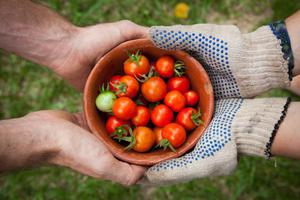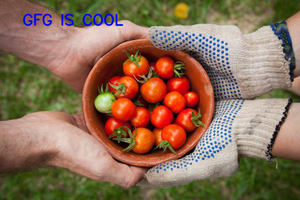Open in App
Not now

# Java Program to Add Text to an image in OpenCV

• Last Updated : 24 Nov, 2020

OpenCV is the cross-platform open-source library for computer vision, image processing, and machine learning.  It plays a major role nowadays in real-time operations in improving modules provides adequate functions for image manipulation. It has C++, C, Python, and Java interfaces and supports Windows, Linux, macOS, iOS, and Android. By using it, one can even process images and videos to identify objects, faces, or even handwriting of a human

Here, putText() which is an inbuilt method will be used after importing this method from its respective module given below in the java program.

Syntax: to import module to deal with images:

`import org.opencv.imgproc.Imgproc;`

Syntax: to use putText() method of this class is as follows:

```putText(image, text, org, fontType, fontSize, color, thickness)
```

Parameters:

Exception: No exception is thrown by this method of this class because simply the tuple is passed. For example in BGR color spectrum, the blue light tuple is as follows: (255,0,0)

Implementation: Input image is as follows been randomly taken. Now text- “GFG IS COOL” is to be added over this image.Input Image

## Java

 `// Importing all OpenCV files``import` `org.opencv.*;``import` `org.opencv.imgproc.Imgproc;`` ` `public` `class` `GFG {`` ` `    ``// Main driver code``    ``public` `static` `void` `main(String args[]) ``throws` `Exception``    ``{`` ` `        ``// Loading the OpenCV core library``        ``System.loadLibrary(Core.NATIVE_LIBRARY_NAME);`` ` `        ``// Reading the contents of the image``        ``// from local computer directory``        ``String src = ``"D:\\InputImage.jpg"``;`` ` `        ``// Creating a Mat object``        ``Mat image = Imgcodecs.imread(src);`` ` `        ``// Text to be added``        ``String text = ``"GFG IS COOL"``;`` ` `        ``// Points from where text should be added``        ``Point org = ``new` `Point(``170``, ``280``);`` ` `        ``// Color of the text``        ``Scalar color = ``new` `Scalar(``0``, ``0``, ``255``);`` ` `        ``// Fonttype of the text to be added``        ``int` `fontType = Imgproc.FONT_HERSHEY_PLAIN;`` ` `        ``// Fontsize of the text to be added``        ``int` `fontSze = ``1``;`` ` `        ``// Thickness of the lines in px``        ``int` `thickness = ``3``;`` ` `        ``// Adding text to the image using putText method``        ``Imgproc.putText(image, text, org, fontType,``                        ``fontSize, color, thickness);`` ` `        ``// Displaying the Image after adding the Text``        ``HighGui.imshow(``""``, image);`` ` `        ``// Waiting for a key event to delay``        ``HighGui.waitKey();``    ``}``}`

Output: The image after adding text is as follows:Output Image

My Personal Notes arrow_drop_up